/

### Calibrating probability distributions used for risk measurement purposes to market-implied data: 1. Introduction

Next Section

1.            Introduction

1.1          Kemp (2005) and Kemp (2009) argue in favour of greater use of market-consistent risk management. This involves greater focus on market implied probability distributions and other risk parameters (consistent with the market prices of derivatives that might protect against the relevant risks) and lesser focus on estimating such parameters using time series analysis of historic market behaviour.

1.2          In practice, as is explained in Kemp (2009), there is insufficient market implied data available to be able to build up a full specification of the relevant market implied probability distribution. However, there is often some market implied data that we can use for calibration purposes. We explain in these pages how we can combine this data with an assumed prior distribution in a Bayesian or credibility-weighted type manner, thereby deriving risk measures that are as market consistent as possible, but still coloured by our prior views in cases where market implied data does not exist. A natural prior to adopt in this context, if we think the historic data is relevant, is one based on the relevant historic dataset.

1.3          A prototype methodology for market consistent risk measurement is described in Kemp (2009) involving an analytical weighted Monte Carlo, aka analytical relative entropy approach. This involves identifying a distribution that is, in some suitable sense, as ‘close as possible’ to the original prior distribution but that has characteristics that match calibration characteristics derived from market implied data (or otherwise).

1.4          As its name suggests, the analytical weighted Monte Carlo methodology has its genesis in the weighted Monte Carlo simulation approach, see Elices & Gimenez (2006) and Avellandeda et al (2001). In this approach, we calibrate a simulation exercise not by changing the draws from some previously specified prior probability distribution but by changing the assumed likelihoods ascribed to each draw in the computation of statistics in which we are interested (e.g. mean, standard deviation, other moments, quantiles etc.). Typically we choose these revised likelihoods of occurrence to minimise the relative entropy between the original prior distribution and the calibrated output distribution. The relative entropy between two discrete distributions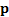and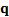is given by the following, wherehere indexes the possible outcomes (the formula for continuous distributions is derived in an analogous manner):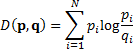1.5          The analytical weighted Monte Carlo methodology notes that in the limit where the number of simulations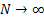we will recover an exact output probability distribution and that in a number of cases of interest this probability distribution can be expressed in ‘analytical’ form, i.e. we may be able to recover relatively simply what the methodology would have produced had we been able to use an infinitely large Monte Carlo sample size.

1.6          In the special case of single instrument calibration involving a univariate normal prior distribution, say,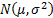we find, as we might expect, that application of an analytical weighted Monte Carlo returns a normal distribution with the following characteristics, see Kemp (2009):

(a)    If we have no market data whatsoever to calibrate to then the calibrated distribution is the same as the prior distribution, i.e.;

(b)   If we have just a mean to calibrate to, say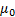, then the calibrated distribution is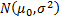; and

(c)    If we have both a mean and a variance to calibrate to, say,and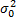, then the calibrated distribution is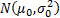.

For risk management purposes, it is usual to adopt the assumption that the return on all assets is the same (i.e. to discount the possibility of ‘manager skill’, because it is prudent to do so). It can be argued that (b) provides the theoretical justification for this, i.e. in effect we are ‘calibrating’ some assumed prior distribution (that might include differential returns) to fit a constraint that requires any prior assumed manager skill in asset selection not actually to be present in practice.Without loss of generality the mean return can for the purposes of this paper be set to zero (since our focus will be on relative returns). Hence our focus will be on second and higher moments (or just second moments in the case of normally distributed variables, which is the main focus of these pages).